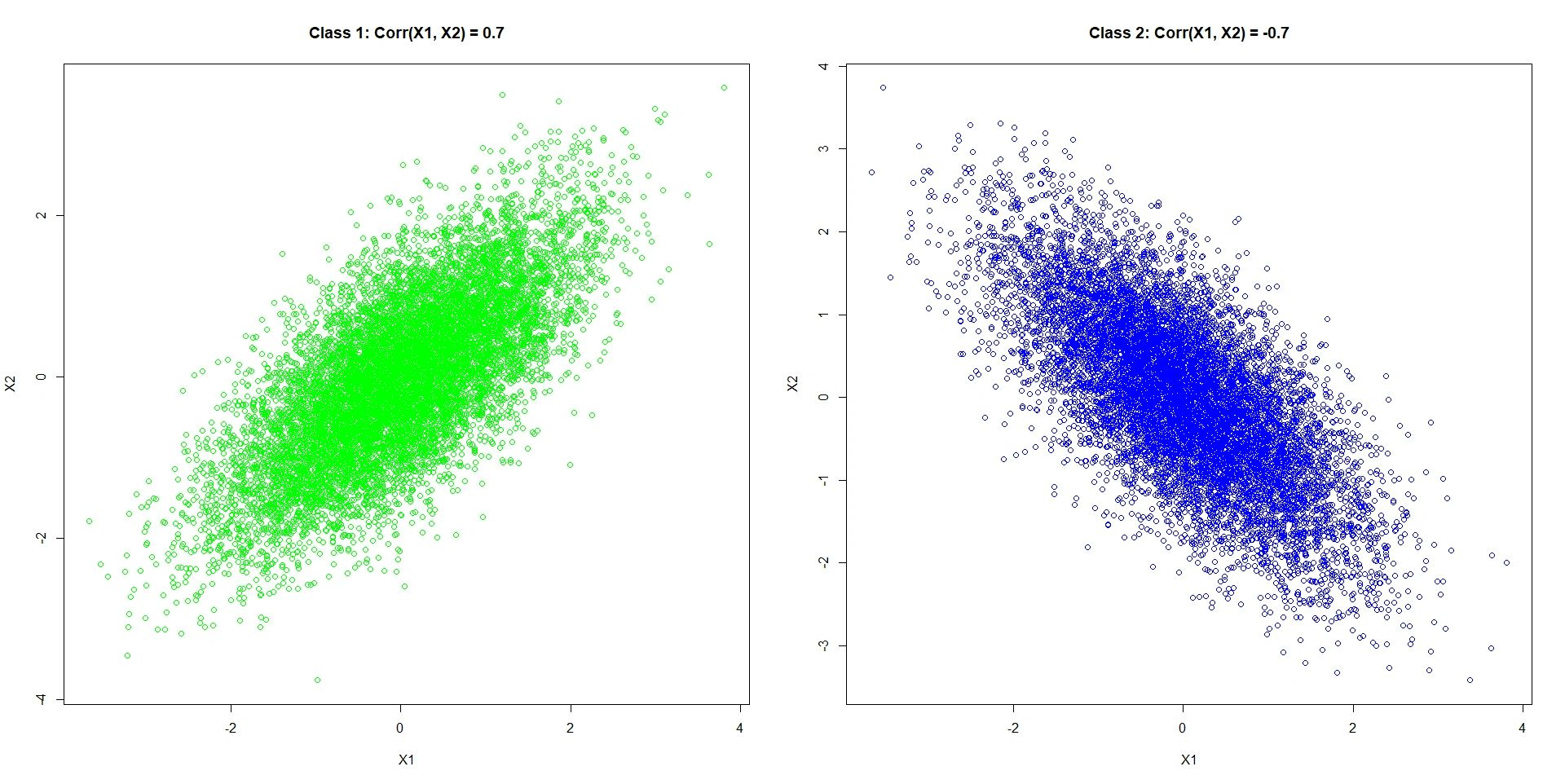Statistical & Financial Consulting by Stanford PhD
NAIVE BAYES

Naive Bayes is a classification method. Suppose the data are split into classes and we observe variables We conveniently assume that in each class the variables are independent (hence the term "naive"). We estimate densities of the continuous variables and probability mass functions of the discrete variables as in each class Then, whenever we see a data point with unknown class membership and known we apply the Bayes rule. We estimate the probability of class membership as

where are unconditional probabilities of each class. The unconditional probabilities can be estimated as the sample frequencies or stipulated using some prior knowledge. The data point is classified to class for which probability (1) is highest.

Naive Bayes performs well in many settings but fails completely in situations where the classes are distinguished by the codependency structure of An example is visualized below.Here the two variables and are normally distributed with mean 0 and standard deviation 1. The only thing which is different between the classes is how the variables relate to each other.

NAIVE BAYES REFERENCES

Barber, D. (2014). Bayesian Reasoning and Machine Learning. Cambridge University Press.

Han, J., Kamber, M. & Pei, J. (2012). Data mining: Concepts and Techniques (3rd ed). Morgan-Kaufman.

Hastie, T., Tibshirani, R., & Friedman, J. H. (2008). The elements of statistical learning: Data mining, inference, and prediction. New York: Springer.

Bishop, C. M. (2006) Pattern Recognition and Machine Learning. New York: Springer.

Witten, I. H., Frank, E., Hall, M., A., & Pal, C. J. (2017). Data Mining: Practical Machine Learning Tools and Techniques (4th ed). New York: Morgan-Kaufmann.

Duda, R. O., Hart, P. E., & Stork, D. G. (2000). Pattern Classification (2nd ed). New York: Wiley-Interscience.

BACK TO THE
STATISTICAL ANALYSES DIRECTORY